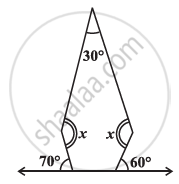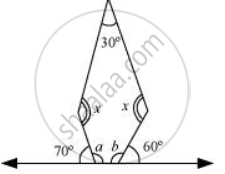# Find the Angle Measure X In the Given Figure - 3 - Mathematics

Find the angle measure x in the given Figure#### SolutionFrom the figure, it can be concluded that,

70 + a = 180° (Linear pair)

a = 110°

60° + b = 180° (Linear pair)

b = 120°

Sum of the measures of all interior angles of a pentagon is 540º.

Therefore, in the given pentagon,

120° + 110° + 30° + x + x = 540°

260° + 2x = 540°

2x = 280°

x = 140°

Concept: Angle Sum Property of a Quadrilateral
Is there an error in this question or solution?

#### APPEARS IN

NCERT Class 8 Maths